# 32. Recursion¶

A child couldn't sleep, so her mother told her a story about a little frog,
who couldn't sleep, so the frog's mother told her a story about a little bear,
who couldn't sleep, so the bear's mother told her a story about a little weasel...
who fell asleep.
...and the little bear fell asleep;
...and the little frog fell asleep;
...and the child fell asleep.


Recursion is an object or process that is defined in terms of itself. Mathematical patterns such as factorials and the Fibonacci series are recursive. Documents that can contain other documents, which themselves can contain other documents, are recursive. Fractal images, and even certain biological processes are recursive in how they work.

## 32.1. Where is Recursion Used?¶

Documents, such as web pages, are naturally recursive. For example, Figure 20.1 shows a simple web document.Figure 20.1: Web page

That web document can be contained in a “box,” which can help layout the page as shown in Figure 20.2.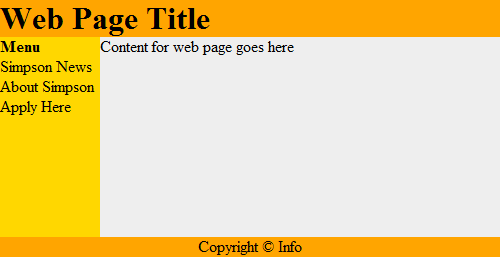Figure 20.2: Web page with tables

This works recursively. Each box can contain a web page, that can have a box, which could contain another web page as shown in Figure 20.3.Figure 20.3: Web page with recursion

Recursive functions are often used with advanced searching and sorting algorithms. We’ll show some of that here and if you take a “data structures” class you will see a lot more of it.

Even if a person does not become a programmer, understanding the concept of recursive systems is important. If there is a business need for recursive table structures, documents, or something else, it is important to know how to specify this to the programmer up front.

For example, a person might specify that a web program for recipes needs the ability to support ingredients and directions. A person familiar with recursion might state that each ingredient could itself be a recipes with other ingredients (that could be recipes.) The second system is considerably more powerful.

## 32.2. How is Recursion Coded?¶

In prior chapters, we have used functions that call other functions. For example:

Functions calling other functions
1def f():
2    g()
3    print("f")
4
5def g():
6    print("g")
7
8f()


It is also possible for a function to call itself. A function that calls itself is using a concept called recursion. For example:

Recursion
1def f():
2    print("Hello")
3    f()
4
5f()


The example above will print Hello and then call the f() function again. Which will cause another Hello to be printed out and another call to the f() function. This will continue until the computer runs out of something called stack space. When this happens, Python will output a long error that ends with:

RuntimeError: maximum recursion depth exceeded

The computer is telling you, the programmer, that you have gone too far down the rabbit hole.

## 32.3. Controlling Recursion Depth¶

To successfully use recursion, there needs to be a way to prevent the function from endlessly calling itself over and over again. The example below counts how many times it has been called, and uses an if statement to exit once the function has called itself ten times.

Controlling recursion levels
 1def f(level):
2    # Print the level we are at
3    print("Recursion call, level",level)
4    # If we haven't reached level ten...
5    if level < 10:
6        # Call this function again
7        # and add one to the level
8        f(level+1)
9
10# Start the recursive calls at level 1
11f(1)

Output
 1Recursion call, level 1
2Recursion call, level 2
3Recursion call, level 3
4Recursion call, level 4
5Recursion call, level 5
6Recursion call, level 6
7Recursion call, level 7
8Recursion call, level 8
9Recursion call, level 9
10Recursion call, level 10


## 32.4. Recursion In Mathematics¶

### 32.4.1. Recursion Factorial Calculation¶

Any code that can be done recursively can be done without using recursion. Some programmers feel that the recursive code is easier to understand.

Calculating the factorial of a number is a classic example of using recursion. Factorials are useful in probability and statistics. For example:

Recursively, this can be described as:

Below are two example functions that calculate . The first one is non-recursive, the second is recursive.

Non-recursive factorial
1# This program calculates a factorial
2# WITHOUT using recursion
3def factorial_nonrecursive(n):
5    for i in range(2, n + 1):

Recursive factorial
1# This program calculates a factorial
2# WITH recursion
3def factorial_recursive(n):
4    if n == 1:
5        return 1
6    elif n > 1:
7        return n * factorial_recursive(n - 1)


The functions do nothing by themselves. Below is an example where we put it all together. This example also adds some print statements inside the function so we can see what is happening.

Trying out recursive functions
 1# This program calculates a factorial
2# WITHOUT using recursion
3
4def factorial_nonrecursive(n):
6    for i in range(2, n + 1):
10
11print("I can calculate a factorial!")
12user_input = input("Enter a number:")
13n = int(user_input)
16
17# This program calculates a factorial
18# WITH recursion
19
20def factorial_recursive(n):
21    if n == 1:
22        return 1
23    else:
24        x = factorial_recursive(n - 1)
25        print( n, "*", x, "=", n * x )
26        return n * x
27
28print("I can calculate a factorial!")
29user_input = input("Enter a number:")
30n = int(user_input)

Output
 1I can calculate a factorial!
2Enter a number:7
32 * 1 = 2
43 * 2 = 6
54 * 6 = 24
65 * 24 = 120
76 * 120 = 720
87 * 720 = 5040
95040
10I can calculate a factorial!
11Enter a number:7
122 * 1 = 2
133 * 2 = 6
144 * 6 = 24
155 * 24 = 120
166 * 120 = 720
177 * 720 = 5040
185040


### 32.4.2. Recursive Expressions¶

Say you have a mathematical expression like this:

$$f_{n} = \begin{cases} 6 & \text{if } n = 1, \\ \frac{1}{2}f_{n-1}+4 & \text{if } n > 1. \end{cases}$$

Looks complicated, but it just means that if $$n=1$$ we are working with $$f_{1}$$. That function returns a 6.

For $$f_{2}$$ we return $$\frac{1}{2}f_{1}+4$$.

1def f(n):


Then we need to add that first case:

1def f(n):
2    if n == 1:
3        return 6


See how closely if follows the mathematical notation? Now for the rest:

1def f(n):
2    if n == 1:
3        return 6
4    elif n > 1:
5        return (1 / 2) * f(n - 1) + 4


Converting these types of mathematical expressions to code is straight forward. But we’d better try it out in a full example:

 1def f(n):
2    if n == 1:
3        return 6
4    elif n > 1:
5        return (1 / 2) * f(n - 1) + 4
6
7
8def main():
9    result = f(10)
10    print(result)
11
12
13main()


## 32.5. Recursive Graphics¶

### 32.5.1. Recursive Rectangles¶

Recursion is great to work with structured documents that are themselves recursive. For example, a web document can have a table divided into rows and columns to help with layout. One row might be the header, another row the main body, and finally the footer. Inside a table cell, might be another table. And inside of that can exist yet another table.

Another example is e-mail. It is possible to attach another person’s e-mail to a your own e-mail. But that e-mail could have another e-mail attached to it, and so on.

Can we visually see recursion in action in one of our Pygame programs? Yes! Figure 19.4 shows an example program that draws a rectangle, and recursively keeps drawing rectangles inside of it. Each rectangle is 20% smaller than the parent rectangle. Look at the code. Pay close attention to the recursive call in the recursive_draw function.Figure 20.4: Recursive Rectangles

recursive_rectangles.py
 1"""
2Recursive Rectangles
3"""
5
6SCREEN_WIDTH = 800
7SCREEN_HEIGHT = 500
8
9
10def draw_rectangle(x, y, width, height):
11    """ Recursively draw a rectangle, each one a percentage smaller """
12
13    # Draw it
15
16    # As long as we have a width bigger than 1, recursively call this function with a smaller rectangle
17    if width > 1:
18        # Draw the rectangle 90% of our current size
19        draw_rectangle(x, y, width * .9, height * .9)
20
21
23    """ Main application class. """
24
25    def __init__(self, width, height):
26        super().__init__(width, height)
27
29
30    def on_draw(self):
31        """ Render the screen. """
33
34        # Find the center of our screen
35        center_x = SCREEN_WIDTH / 2
36        center_y = SCREEN_HEIGHT / 2
37
38        # Start our recursive calls
39        draw_rectangle(center_x, center_y, SCREEN_WIDTH, SCREEN_HEIGHT)
40
41
42def main():
43
44    MyWindow(SCREEN_WIDTH, SCREEN_HEIGHT)
46
47
48if __name__ == "__main__":
49    main()


### 32.5.2. Fractals¶

Fractals are defined recursively. Here is a very simple fractal, showing how it changes depending on how “deep” the recursion goes.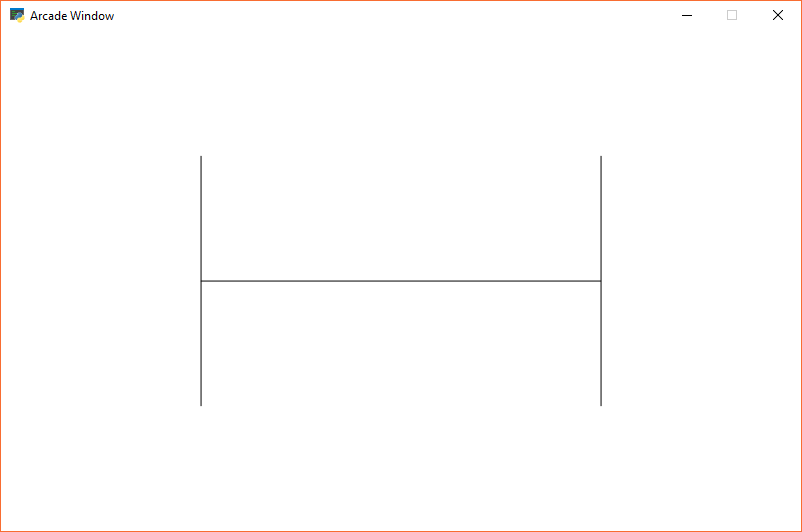Figure 20.5: Recursive Fractal Level 0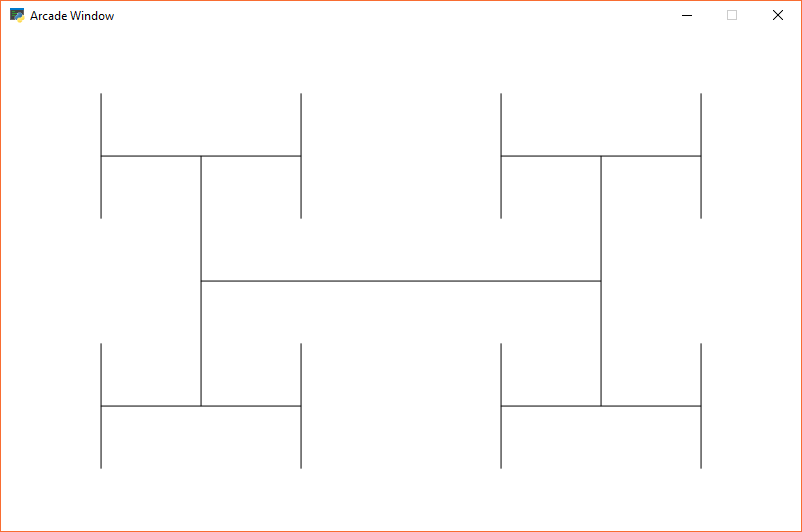Figure 20.6: Recursive Fractal Level 1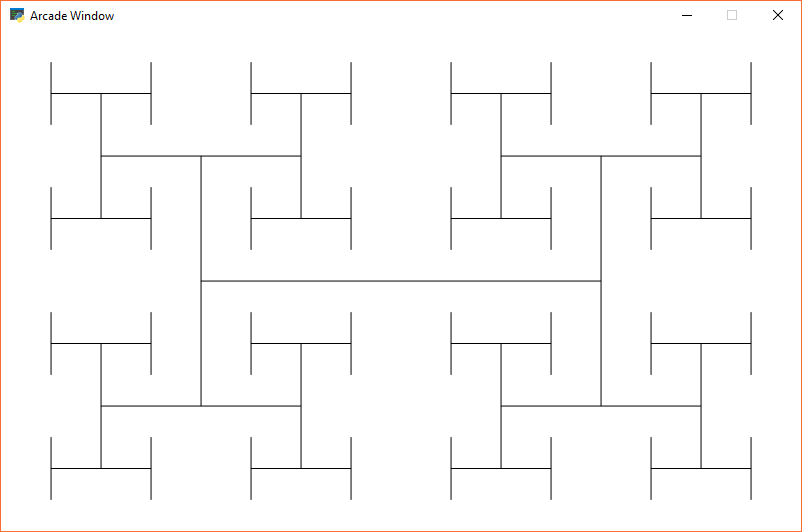Figure 20.7: Recursive Fractal Level 2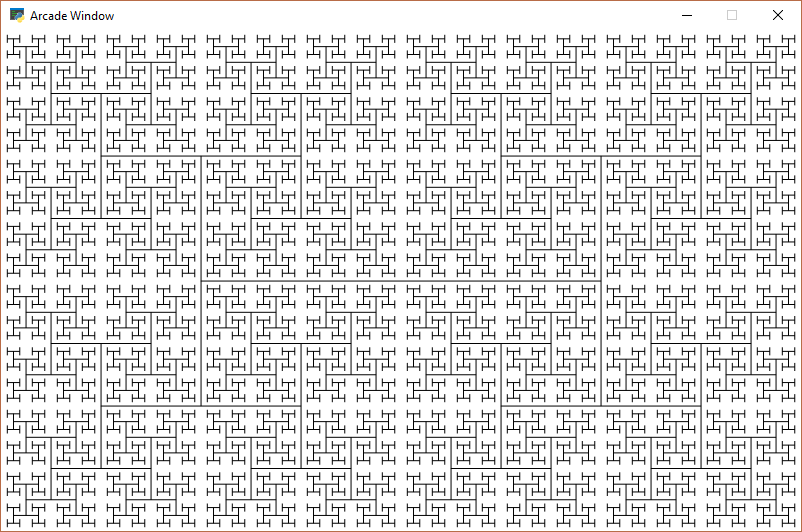Figure 20.8: Recursive Fractal Level 5

Here is the source code for the “H” fractal:

recursive_h.py
 1"""
2Recursive H's
3"""
5
6SCREEN_WIDTH = 800
7SCREEN_HEIGHT = 500
8
9RECURSION_DEPTH = 0
10
11
12def draw_h(x, y, width, height, count):
13    """ Recursively draw an H, each one a half as big """
14
15    # Draw the H
16    # Draw cross-bar
17    arcade.draw_line(x + width * .25, height / 2 + y,
18                     x + width * .75, height / 2 + y, arcade.color.BLACK)
19    # Draw left side
20    arcade.draw_line(x + width * .25, height * .5 / 2 + y,
21                     x + width * .25, height * 1.5 / 2 + y, arcade.color.BLACK)
22    # Draw right side
23    arcade.draw_line(x + width * .75, height * .5 / 2 + y,
24                     x + width * .75, height * 1.5 / 2 + y, arcade.color.BLACK)
25
26    # As long as we have a width bigger than 1, recursively call this function with a smaller rectangle
27    if count > 0:
28        count -= 1
29        # Draw the rectangle 90% of our current size
30        # Draw lower left
31        draw_h(x, y, width / 2, height / 2, count)
32        # Draw lower right
33        draw_h(x + width / 2, y, width / 2, height / 2, count)
34        # Draw upper left
35        draw_h(x, y + height / 2, width / 2, height / 2, count)
36        # Draw upper right
37        draw_h(x + width / 2, y + height / 2, width / 2, height / 2, count)
38
39
41    """ Main application class. """
42
43    def __init__(self, width, height):
44        super().__init__(width, height)
45
47
48    def on_draw(self):
49        """ Render the screen. """
51
52        # Start our recursive calls
53        draw_h(0, 0, SCREEN_WIDTH, SCREEN_HEIGHT, RECURSION_DEPTH)
54
55
56def main():
57    MyWindow(SCREEN_WIDTH, SCREEN_HEIGHT)
59
60
61if __name__ == "__main__":
62    main()


You can explore fractals on-line:

If you want to program your own fractals, you can get ideas of easy fractals by looking at Chapter 8 of The Nature of Code by Daniel Shiffman.

## 32.6. Recursive Mazes¶

There are maze generation algorithms. Wikipedia has a nice Maze generation algorithm article that details some. One way is the recursive division method.

The algorithm is described below. Images are from Wikipedia.Begin with the maze’s space with just the outside walls. Call this a chamber.Divide the chamber with one random horizontal wall, and one random vertical wall.Pick three of the four walls and put a randomly position opening in it.Subdivide each of the four sections. Call each a chamber. Recursively call this function. In this image, the top left chamber has been subdivided.

This method results in mazes with long straight walls crossing their space, making it easier to see which areas to avoid.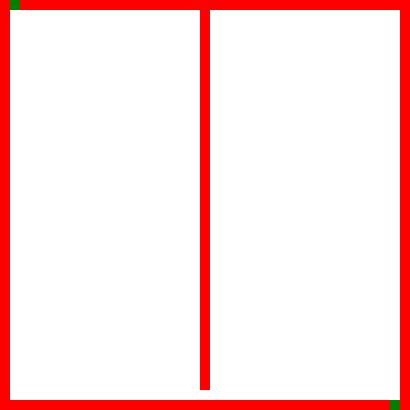Recursive maze generation. Source: Wikipedia Maze generation algorithm.

Here is sample Python code that creates a maze using this method:

Recursive Maze Example
  1import random
2
3# These constants are used to determine what should be stored in the grid if we have an empty
4# space or a filled space.
5EMPTY = "   "
6WALL = "XXX"
7
8# Maze must have an ODD number of rows and columns.
9# Walls go on EVEN rows/columns.
10# Openings go on ODD rows/columns
11MAZE_HEIGHT = 51
12MAZE_WIDTH = 51
13
14
15def create_grid(width, height):
16    """ Create an empty grid. """
17    grid = []
18    for row in range(height):
19        grid.append([])
20        for column in range(width):
21            grid[row].append(EMPTY)
22    return grid
23
24
25def print_maze(maze):
26    """ Print the maze. """
27
28    # Loop each row, but do it in reverse so 0 is at the bottom like we expect
29    for row in range(len(maze) - 1, -1, -1):
30        # Print the row/y number
31        print(f"{row:3} - ", end="")
32
33        # Loop the row and print the content
34        for column in range(len(maze[row])):
35            print(f"{maze[row][column]}", end="")
36
37        # Go down a line
38        print()
39
40    # Print the column/x at the bottom
41    print("     ", end="")
42    for column in range(len(maze)):
43        print(f"{column:3}", end="")
44    print()
45
46
47def create_outside_walls(maze):
48    """ Create outside border walls."""
49
50    # Create left and right walls
51    for row in range(len(maze)):
52        maze[row] = WALL
53        maze[row][len(maze[row])-1] = WALL
54
55    # Create top and bottom walls
56    for column in range(1, len(maze) - 1):
57        maze[column] = WALL
58        maze[len(maze) - 1][column] = WALL
59
60
61def create_maze(maze, top, bottom, left, right):
62    """
63    Recursive function to divide up the maze in four sections
64    and create three gaps.
65    Walls can only go on even numbered rows/columns.
66    Gaps can only go on odd numbered rows/columns.
67    Maze must have an ODD number of rows and columns.
68    """
69
70    # Figure out where to divide horizontally
71    start_range = bottom + 2
72    end_range = top - 1
73    y = random.randrange(start_range, end_range, 2)
74
75    # Do the division
76    for column in range(left + 1, right):
77        maze[y][column] = WALL
78
79    # Figure out where to divide vertically
80    start_range = left + 2
81    end_range = right - 1
82    x = random.randrange(start_range, end_range, 2)
83
84    # Do the division
85    for row in range(bottom + 1, top):
86        maze[row][x] = WALL
87
88    # Now we'll make a gap on 3 of the 4 walls.
89    # Figure out which wall does NOT get a gap.
90    wall = random.randrange(4)
91    if wall != 0:
92        gap = random.randrange(left + 1, x, 2)
93        maze[y][gap] = EMPTY
94
95    if wall != 1:
96        gap = random.randrange(x + 1, right, 2)
97        maze[y][gap] = EMPTY
98
99    if wall != 2:
100        gap = random.randrange(bottom + 1, y, 2)
101        maze[gap][x] = EMPTY
102
103    if wall != 3:
104        gap = random.randrange(y + 1, top, 2)
105        maze[gap][x] = EMPTY
106
107    # Print what's going on
108    print(f"Top/Bottom: {top}, {bottom} Left/Right: {left}, {right} Divide: {x}, {y}")
109    print_maze(maze)
110    print()
111
112    # If there's enough space, to a recursive call.
113    if top > y + 3 and x > left + 3:
114        create_maze(maze, top, y, left, x)
115
116    if top > y + 3 and x + 3 < right:
117        create_maze(maze, top, y, x, right)
118
119    if bottom + 3 < y and x + 3 < right:
120        create_maze(maze, y, bottom, x, right)
121
122    if bottom + 3 < y and x > left + 3:
123        create_maze(maze, y, bottom, left, x)
124
125
126def main():
127
128    # Create the blank grid
129    maze = create_grid(MAZE_WIDTH, MAZE_HEIGHT)
130
131    # Fill in the outside walls
132    create_outside_walls(maze)
133
134    # Start the recursive process
135    create_maze(maze, MAZE_HEIGHT - 1, 0, 0, MAZE_WIDTH - 1)
136
137
138if __name__ == "__main__":
139    main()